Built-In Self Test (BIST) for Embedded Systems - 2

# Built-In Self Test (BIST) for Embedded Systems - 2 Notes | Study Embedded Systems (Web) - Computer Science Engineering (CSE)

## Document Description: Built-In Self Test (BIST) for Embedded Systems - 2 for Computer Science Engineering (CSE) 2022 is part of Embedded Systems (Web) preparation. The notes and questions for Built-In Self Test (BIST) for Embedded Systems - 2 have been prepared according to the Computer Science Engineering (CSE) exam syllabus. Information about Built-In Self Test (BIST) for Embedded Systems - 2 covers topics like and Built-In Self Test (BIST) for Embedded Systems - 2 Example, for Computer Science Engineering (CSE) 2022 Exam. Find important definitions, questions, notes, meanings, examples, exercises and tests below for Built-In Self Test (BIST) for Embedded Systems - 2.

Introduction of Built-In Self Test (BIST) for Embedded Systems - 2 in English is available as part of our Embedded Systems (Web) for Computer Science Engineering (CSE) & Built-In Self Test (BIST) for Embedded Systems - 2 in Hindi for Embedded Systems (Web) course. Download more important topics related with notes, lectures and mock test series for Computer Science Engineering (CSE) Exam by signing up for free. Computer Science Engineering (CSE): Built-In Self Test (BIST) for Embedded Systems - 2 Notes | Study Embedded Systems (Web) - Computer Science Engineering (CSE)
 1 Crore+ students have signed up on EduRev. Have you?

Ones Count

The number of ones in the CUT output response is counted. In this method the number of ones is the signature. It requires a simple counter to accomplish the goal. Figure 40.10 shows the test structure of ones count for a single output CUT. For multiple output ones, a counter for each output or one output at a time with the same input sequence can be used. Input test sequence can be permuted without changing the count.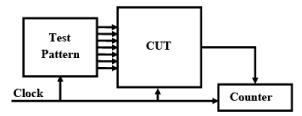Fig. 40.10 Ones count compression circuit structure

For N-bit test length with r ones the masking probability is shown as follows:

Number of masking sequences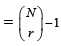2possible output sequences with only one fault free.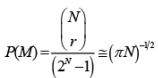It has low masking probability for very small and very large r. It always detects odd number of errors and it may detect even number of errors.

Transition Count

It is very similar to ones count technique. In this method the number of transitions in the CUT response, zero to one and/or one to zero is counted. Figure 40.11 shows a test structure of transition counting. It has simple hardware DFF with EXOR to detect a transition and counter to count number of transitions. It has less aliasing probability than ones counting. Test sequences cannot be permuted. Permutation of input sequences will change the number of transitions. On the other hand, one can reorder the test sequence to maximize or minimize the transitions, hence, minimize the aliasing probability.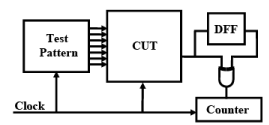Fig. 40.11 Transition count compression circuit structure

For N-bit test length with r transitions the masking probability is shown as follows: For the test length of N, there are N-1 transitions.

Number of masking sequencesHence,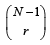is the number of sequences that has r transitions.

Since the first output can be either one or zero, therefore, the total number must be multiplied by

2. Therefore total number of sequences with same transition counts :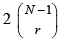. Again, only one of them is fault-free.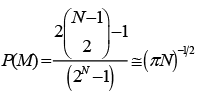Syndrome Testing

Syndrome is defined as the probability of ones of the CUT output response. The syndrome is 1/8 for a 3-input AND gate and 7/8 for a 3-input OR gate if the inputs has equal probability of ones and zeros. Figure 40.12 shows a BIST circuit structure for the syndrome count. It is very similar to ones count and transition count. The difference is that the final count is divided by the number of patterns being applied. The most distinguished feature of syndrome testing is that the syndrome is independent of the implementation. It is solely determined by its function of the circuit.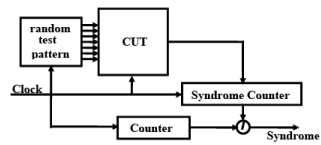Fig. 40.12 Syndrome testing circuit structure

The originally design of syndrome test applies exhaustive patterns. Hence, the syndrome is S = K /2n , where n is the number of inputs and K is the number of minterms. A circuit is syndrome testable if all single stuck-at faults are syndrome detectable. The interesting part of syndrome testing is that any function can be designed as being syndrome testable.

LFSR Structure

• External and internal type LFSR is used. Both types use D type flip-flop and exclusiveOR logic as shown in Figure 40.13.
• In external type LFSR, XOR gates are placed outside the shift path. It is also called type 1 LFSR .
• In internal type LFSRs, also called type 2 LFSR, XOR gates are placed in between the flip-flops.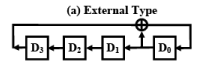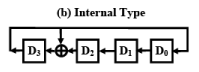Fig. 40.13 Two types of LFSR

One of the most important properties of LFSRs is their recurrence relationship. The recurrence relation guarantees that the states of a LFSR are repeated in a certain order. For a given sequence of numbers a0, a1, a2,…………an,…….. We can define a generating function:

G(x) = a0 + a1x + a2x2 + …………+ amxm + ……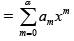m } = {α012}

where αior 0 depending on the out put stage and time ti.

The initial states are a-n, a-n+1,…….,a-2, a-1. The recurrent relation defining {am}is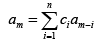where ci=0, means output is not fed back

=1,otherwise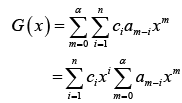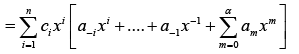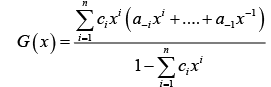G(x) has been expressed in terms of the initial state and the feedback coefficients. The denominator of the polynomial G(x), f (x) =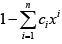is called the characteristic polynomial of  the LFSR.

LFSR for Response Compaction: Signature Analysis

• It uses cyclic redundancy check code (CRCC) generator (LFSR) for response compacter
• In this method, data bits from circuit Pos to be compacted as a decreasing order coefficient polynomial
• CRCC divides the PO polynomial by its characteristic polynomial that leaves remainder of division in LFSR. LFSR must be initialized to seed value (usually 0) before testing.
• After testing, signature in LFSR is compared to known good machine signature

For an output sequence of length N, there is a total of 2N-1 faulty sequence. Let the input sequence is represented as P(x) as P(x)=Q(X)G(x)+R(x). G(x) is the characteristic polynomial; Q(x) is the quotient; and R(x) is the remainder or signature. For those aliasing faulty sequence, the remainder R(x) will be the same as the fault-free one. Since, P(x) is of order N and G(x) is of order r, hence Q(x) has an order of N-r. Hence, there are 2N-r possible Q(x) or P(x). One of them is fault-free. Therefore, the aliasing probability is shown as follows: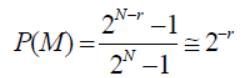for large N. Masking probabilities is independent of input sequence. Figure 40.14 illustrates a modular LFSR as a response compactor.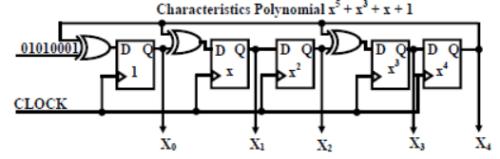Fig. 40.14 Modular LFSR as a response compactor

• Any divisor polynomial G(x) with two or more non-zero coefficients will detect all single-bit errors.

Multiple-Input Signature Register (MISR)

• The problem with ordinary LFSR response compacter is too much hardware overhead if one of these is put on each primary output (PO).
• Multiole-input signature register (MISR) is the solution that compacts all outputs into one LFSR. It works because LFSR is linear and obeys superposition principle.
• All responses are superimposed in one LFSR. The final remainder is XOR sum of remainders of polynomial divisions of each PO by the characteristic polynomial.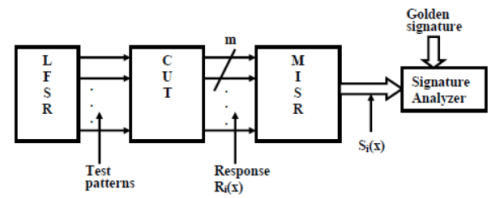Fig. 40.15 Multiple input signature register

Figure 40.15 illustrates a m-stage MISR. After test cycle i, the test responses are stable on CUT outputs, but the shifting clock has not yet been applied.

Ri(x)= (m-1)th polynomial representing the test responses after test cycle i.

Si(x)=polynomial representing the state of the MISR after test cycle i.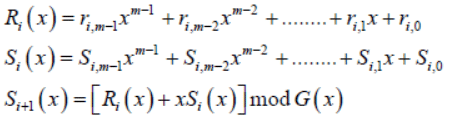G (x) is thecharacteristicpolynomial

Assume initial state of MISR is 0. So,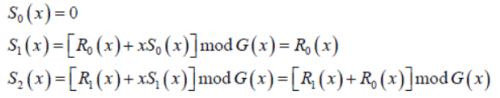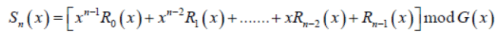This is the signature left in MISR after n patterns are applied. Let us consider a n-bit response compactor with m-bit error polynomial. Then the error polynomial is of (m+n-2) degree that gives (2m+n-1-1) non-zero values. G(x) has 2n-1-1 nonzero multiples that result m polynomials of degree <=m+n-2.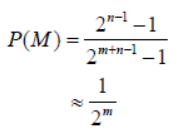The document Built-In Self Test (BIST) for Embedded Systems - 2 Notes | Study Embedded Systems (Web) - Computer Science Engineering (CSE) is a part of the Computer Science Engineering (CSE) Course Embedded Systems (Web).
All you need of Computer Science Engineering (CSE) at this link: Computer Science Engineering (CSE)

## Embedded Systems (Web)

47 videos|69 docs|65 tests
 Use Code STAYHOME200 and get INR 200 additional OFF

## Embedded Systems (Web)

47 videos|69 docs|65 tests

Track your progress, build streaks, highlight & save important lessons and more!

,

,

,

,

,

,

,

,

,

,

,

,

,

,

,

,

,

,

,

,

,

;